# Abstract

This article attempts to model the price of bitcoin as a function of supply and demand. We explore the possibility of a log-log time model for demand and reject it upon the discovery of serious heteroscedasticity. We then find an autoregressive integrated moving average model with adequate performance using the R program auto.arima. The forecast of the demand via the ARIMA is then used to model future bitcoin price, given the known values for the supply.

# Notes

• Minimum prerequisite reading: [1, 2, 6]
• All analysis was performed using Stata 14 and R 3.4.4
• This is not financial advice.
• All models are wrong, some are useful.

# Introduction

The Stock to flow ratio (which we will now abbreviate to St/F for brevity) has been shown to be a non-spurious predictor in determining Bitcoin price [1,2,3].

A common criticism of the St/F model is that it does not allow for the contribution of demand in its value model. After all, price is a function of supply and demand[6,7]. St/F models the supply side of things, but how does it account for changes in demand?

In this article, we will hold two ideas as absolute truth, even though they might not be. We will call these truths — axioms. We will establish these axioms so as to enable a framework from which to further extend the model of supply and demand.

# Notation

Medium is relatively limited for mathematical notation. The usual notation for an estimate of a statistical parameter is to place a hat on top. Instead, we define the estimate of a term as []. e.g. the estimate of β = [β]. If we are representing a 2x2 matrix, we will do so like this [r1c1, r1c2 \ r2c1, r2c2] etc. Subscripted items are superseded by @ — eg for the 10th position in a vector X we would normally subscript X with 10. We will instead write X@10.

# Axiom 1: Price is a function of supply and demand

Quoting from Wiki :

In microeconomics, supply and demand is an economic model of price determination in a market. It postulates that, holding all else equal, in a competitive market, the unit price for a particular good, or other traded item such as labor or liquid financial assets, will vary until it settles at a point where the quantity demanded (at the current price) will equal the quantity supplied (at the current price), resulting in an economic equilibrium for price and quantity transacted.

Let us assume the following: price (P)= demand (D)/supply (S) The higher the supply, if the demand remains constant, then the lower the price. The higher the demand D, if the supply remains constant, the higher the price.

Here we define flow as the monthly flow, so as to not confuse long run effects. Now let us assume that the supply side of bitcoin is modelled by the inverse of the scarcity (i.e. abundance), i.e. S= 1/St/F +ε = F/St+ε, where ε is some random error. Our equation is then price P= D/(F/St+ε). Let us assume then also, that demand D is some function. We will also assume that ε is i.i.d~Normal(0,1) and thus can be ignored from the model (for now).

Thus we have established that P = D/(F/St), it then follows that D = PF/St

# Axiom 2: Demand is a function of time t

Now let us assert that demand is modelled by some function of time f(t)=D=βt.

Ordinary least squares (OLS) regression is a way to estimate a linear relationship between two or more variables. First, let us define a linear model as some function of X that equals Y with some error.

Y = βX+ε

where Y is the dependent variable, X is the independent variable, ε is the error term and β is the multiplier of X. The goal of OLS is to estimate β such that ε is minimised.

In order for [β] to be a reliable estimate, some basic assumptions must be met:

1. There is a linear relationship between the dependent and independent variables
2. The errors are homoscedastic (that is — they have a constant variance)
3. The error is normally distributed with a mean of zero
4. There is no autocorrelation in the error (that is — the errors aren’t correlated with the lag of the errors)

We can now estimate [D] via the least squares model [D]=[β]t+ε.

## Linearity

We begin by taking a look at the non-transformed scatter plot of demand v time.

In figure 1, we encounter a familiar pattern of exponential growth. This is usually fit well by a log-log model (figure 2).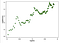figure 2 — a straight line on a log-log graph indicates a good match for exponential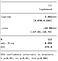figure 3 — bootstrapped log-log regression (using robust estimator for variance)

From figure 3 we see our estimate is log([D])=3.98log(t) -16 , from which we can then infer for each 10% increase in time, we expect about a 46% increase in demand (e.g. 1.10^3.98=1.46).

Using the model, we can now estimate the residuals [ε] and fitted values [Y] and test the other assumptions.

## Homoscedasticity

If the assumption of constant variance in the error term (i.e. homoscedasticity) were to be true, then the error term would vary randomly around 0 for each value in the predicted values. The residuals v fitted (RVF) plot (figure 5) is, therefore, a simple yet effective graphical way to investigate the accuracy of this assumption. In figure 5, we see there is a definite pattern, rather than a random scattering, indicating a there is significant non-constant variance in the error term (i.e. heteroscedasticity).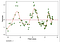Figure 5 — RVF plot. A pattern here indicates there might be an issue.

Heteroscedasticity like this causes the estimates of the coefficients [β] to have much larger variance and thus be less precise and leads to p-values that overstate their significance, because the OLS procedure does not detect the increased variance. Therefore when we then calculate t-values and F values we use an underestimation of the variance, leading to higher (untrue) significance. This also has an effect on the 95% confidence interval about [β], which is itself a function of the variance (via the standard error). To try to improve this situation, the robust sandwich estimator is used in determining the variance and the regression is bootstrapped (this is a form of resampling). However these results indicate that even after these adjustments, we can still not really trust the results of this OLS. Arguably, every OLS model of time and price [i.e. 10] suffers from this problem. Instead, we will investigate another more suitable model of time — the ARIMA model.

## ARIMA

More appropriate than simply regressing time or transformations thereof, ARIMA is a technique that has been developed to model changes of a time series over time. ARIMA is short for Auto Regressive Integrated Moving Averages. It encompasses a whole class of models that explain time series based on their own past values — such as the lags and the lagged forecast errors. Any time series that exhibits patterns and is not random white noise can be modelled with an ARIMA model (or a modified version thereof).

Basic ARIMA models are characterised by three terms: p, d, q.

where,

• p is the order of the autoregressive (AR) term
• q is the order of the moving average term (MA), and
• d is the number of differencing required to make the time series stationary (I)

Using the R program auto.arima from the forecast package[8, 9] we are able to select an ARIMA model that fits the data according to the lowest AIC — the program runs through various combinations of p, q and d and finds the best fit. Here we can see it has selected the autoregressive order of 3, the moving average order of 1 and the order of integration of 2 (interestingly, auto.arima utilises the KPSS test to determine the appropriate order of integration . Readers of  will be familiar with this test).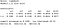Figure 6 — R program auto.arima has automatically selected the best parameters for the ARIMA

In figure 6 we have identified the coefficients for the ARIMA .Figure 7 — Some goodness of fit statistics for the model in figure 6

Now observing the Root Mean Square Error (RMSE) in Figure 7, we expect there to be little difference between the predicted demand and the actual demand. In figure 8 where this is plotted, we can see from a glance that this model estimates previous demand much closer than the OLS.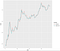Figure 8 — the fitted ARIMA values for log demand — a much better fit than the OLS.

Generating a dynamic forecast from the ARIMA is a little complicated to express in formulas here, but if you do want to see the gory details — take the time to read through [8 & 9].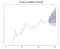Figure 9— ARIMA forecast for log demand over the next 2 years

How does our demand forecast look in the linear scale?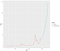Figure 10— demand and demand forecast from the ARIMA in the linear space.

# Connecting the models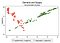Figure 11 — Demand and Supply as a function of price

Now we can plug in our forecast data and the expected stock and flow values to estimate the forecast price.

Previously, we established that P = D/(F/St) see Axiom 1.

We know what flow and stock are going to be (within a close margin) going forward, thus we can plug in those figures and the forecast from our ARIMA of demand. The results are shown in figure 12.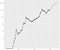Figure 12— the actual price is marked as points, the model price is the line. Here we can see we could reasonably expect a bitcoin price of >\$100,000 by the end of 2021.

# Conclusion

We have presented a simple and relatively parsimonious model of supply and demand for the price of bitcoin, where supply is modelled as the abundance (i.e. the inverse of the scarcity, as measured by stock to flow). There is potential to extend this basic model, in particular by exploring models of demand that are based on other variables rather than time.

# Caveats

• This forecast relies heavily on the ARIMA. The ARIMA could be wrong — lots of models are wrong all the time. They are just a simplification of reality to try to help us understand reality. In this, we are trying to model the price of bitcoin as a function of supply and demand.
• Basically, we have not performed any diagnostic tests on the ARIMA — take it or leave it. The point was to find a way to model price as a function of supply and demand, not to find the best model of supply and demand. This is left as an exercise for the reader.
• Further, Axiom 2 very much might not actually be true. Time might be a good surrogate for the true adoption curve, but it is unlikely that it alone explains demand.
• The whole idea that price is a simple function of supply and demand (i.e. axiom 1) is probably not the whole story(i.e. see ). There are likely to be feedback loops and other structural connections (and emotionality in consumption etc) that are not expressed in this simple equation. Keep an eye out for further development and investigation into potential structural relationships.

# Citations

 Hyndman R, Athanasopoulos G, Bergmeir C, Caceres G, Chhay L, O'Hara-Wild M, Petropoulos F, Razbash S, Wang E, Yasmeen F (2019). forecast: Forecasting functions for time series and linear models. R package version 8.9, http://pkg.robjhyndman.com/forecast.

 Hyndman RJ, Khandakar Y (2008). “Automatic time series forecasting: the forecast package for R.” Journal of Statistical Software, 26(3), 1–22. http://www.jstatsoft.org/article/view/v027i03.

Various other charts etc that enable us to see the supply and demand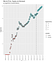Figure A1 — Here is a look at the supply and demand, with the forecast shown after the dotted line. Red circles are supply circles, green are demand. The size of the circles is relative to the size of the supply or demand. Towards the beginning, we see that there isn’t much in the way of demand, but towards the end, demand has picked up and supply (as scheduled) has dropped off.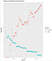Figure A2 — Supply and demand through time — telling much the same story as figure A1.

# Digital Signature

— — -BEGIN BITCOIN SIGNED MESSAGE — — -
A Model of Supply and Demand for Bitcoin Value
— — -BEGIN SIGNATURE — — -
IL9Tlu3rVIDMDM0t15gxQ31WgMrLP/XgaFS4a3Knxef0QrxO06hFgZm0tJwjVdjhpUc/8g29/h9rsgW7T0F8I8I=
— — -END BITCOIN SIGNED MESSAGE — — -

## Coinmonks

#### Coinmonks is a non-profit Crypto educational publication. Follow us on Twitter @coinmonks Our other project — https://coincodecap.com

Welcome to a place where words matter. On Medium, smart voices and original ideas take center stage - with no ads in sight. Watch
Follow all the topics you care about, and we’ll deliver the best stories for you to your homepage and inbox. Explore
Get unlimited access to the best stories on Medium — and support writers while you’re at it. Just \$5/month. Upgrade